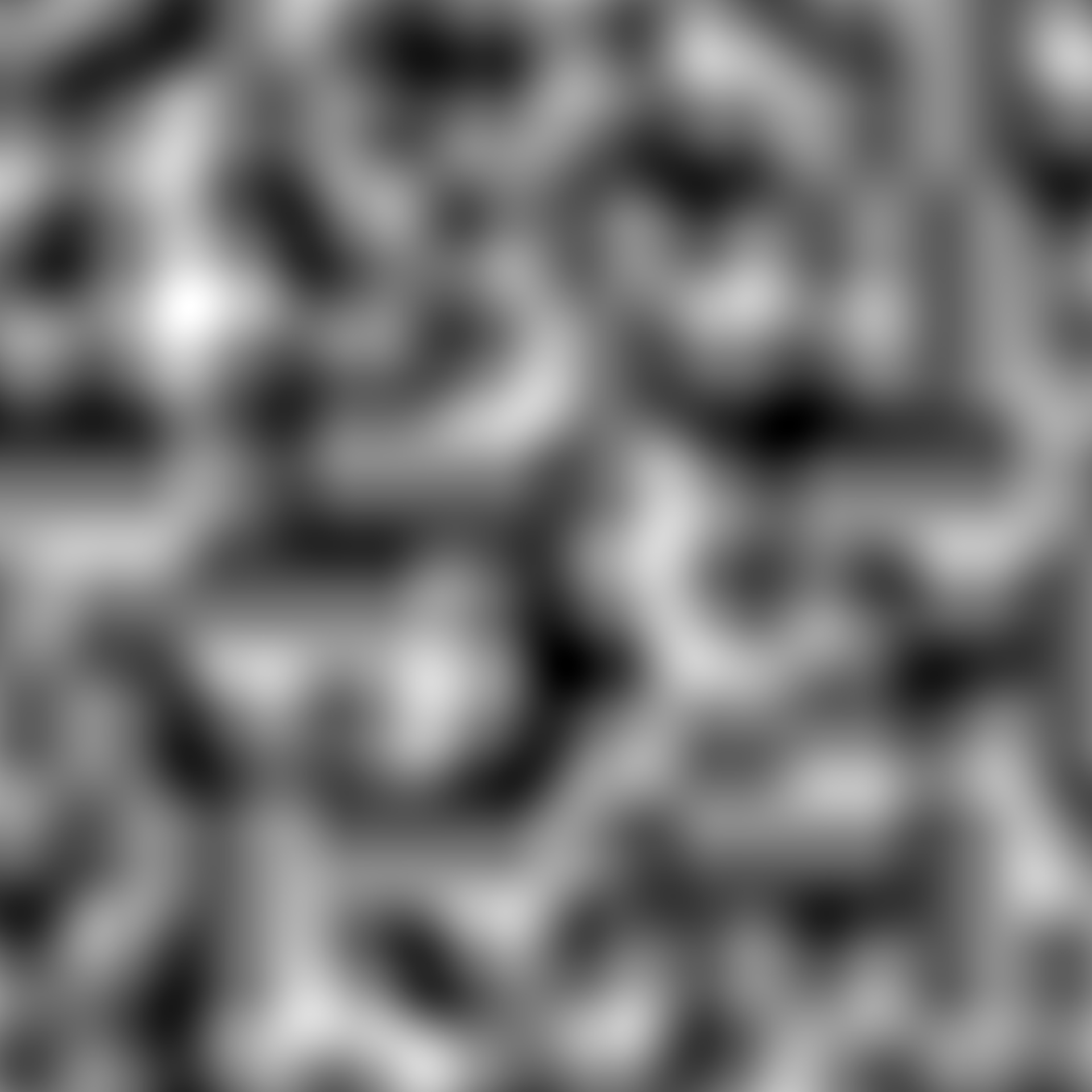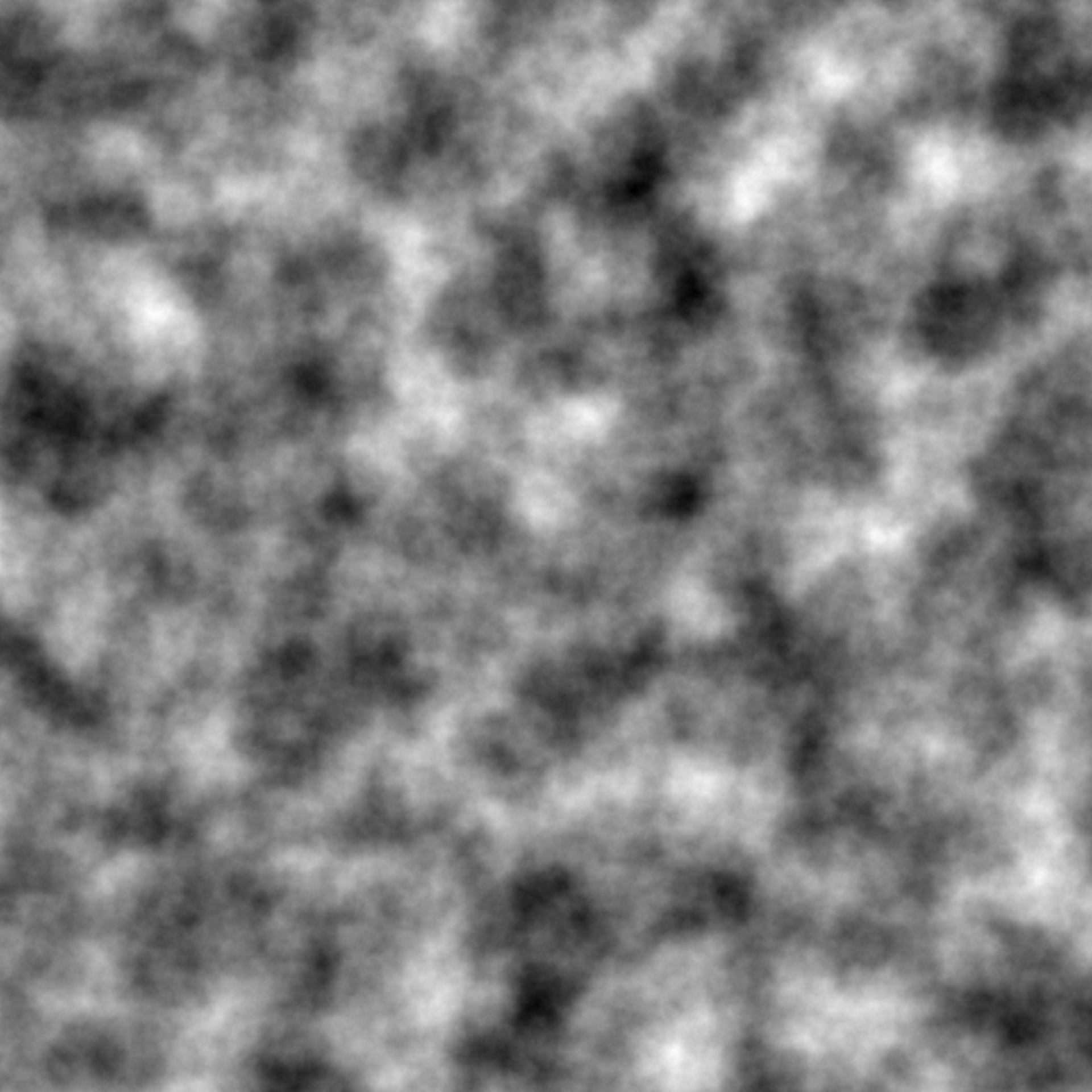This function allows you to create fractals of a given noise or pattern generator by calculating it repeatedly at changing frequency and combining the results based on a fractal function.

fracture(
noise,
fractal,
octaves,
gain = ~./2,
frequency = ~. * 2,
seed = NULL,
...,
fractal_args = list(),
gain_init = 1,
freq_init = 1
)

## Arguments

noise The noise function to create a fractal from. Must have a frequency argument. The fractal function to combine the generated values with. Can be one of the provided ones or a self-made function. If created by hand it must have the following arguments: base: The current noise values new: The new noise values to combine with base strength: The value from gain corresponding to the index of new octave: The index of new And must return a numeric vector of the same length as new The number of generated values to combine The intensity of the generated values at each octave. The interpretation of this is up to the fractal function. Usually the intensity will gradually fall as the frequency increases. Can either be a vector of values or a (lambda) function that returns a new value based on the prior, e.g. ~ . / 2. The default is often a good starting point though e.g. ridged() fractal has been designed with a special gain function. The frequency to use at each octave. Can either be a vector of values or a function that returns a new value based on the prior. See gain. A seed for the noise generator. Will be expanded to the number of octaves so each gets a unique seed. arguments to pass on to generator Additional arguments to fractal as a named list The gain and frequency for the first octave if gain and/or frequency are given as a function.

ambient comes with a range of build in fractal functions: fbm(), billow(), ridged(), clamped()

## Examples

grid <- long_grid(seq(1, 10, length.out = 1000), seq(1, 10, length.out = 1000))

# When noise is generated by it's own it doesn't have fractal properties
grid$clean_perlin <- gen_perlin(grid$x, grid$y) plot(grid, clean_perlin)# Use fracture to apply a fractal algorithm to the noise grid$fractal_perlin <- fracture(gen_perlin, fbm, octaves = 8,
x = grid$x, y = grid$y)
plot(grid, fractal_perlin)